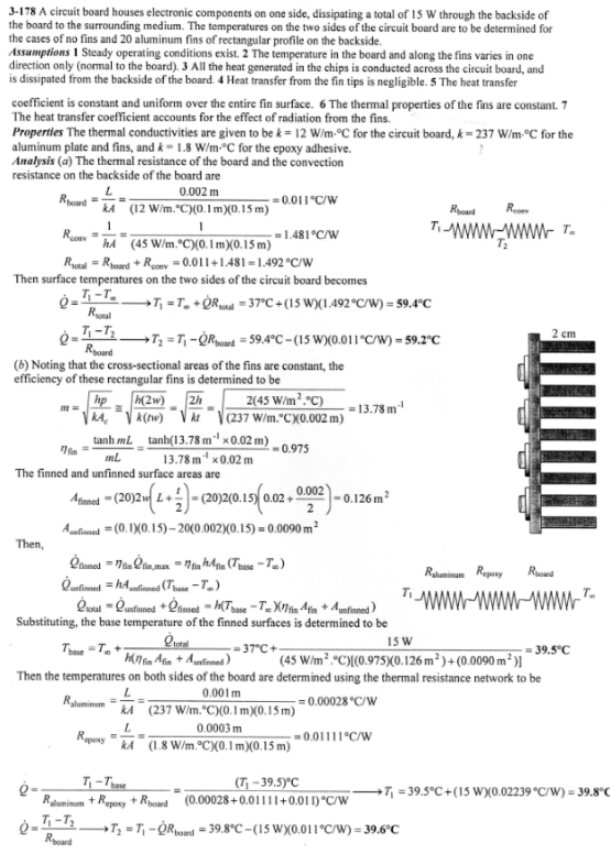heat and mass transfer problems engineering equations heat and mass transfer school homework engineering heat and mass transfer formulas heat and mass transfer solutions to heat and mass transfer problems full solution engineering problem solution heat and mass transfer math problems engineering equations heat and mass transfer school homework engineering solutions to heat and mass transfer formulas heat problems mass problem solutions to transfer problems full solution heat and mass transfer
heat and mass transfer problems engineering equations heat and mass transfer school homework engineering heat and mass transfer formulas heat and mass transfer solutions to heat and mass transfer problems full solution engineering problem solution heat and mass transfer math problems engineering equations heat and mass transfer school homework engineering solutions to heat and mass transfer formulas heat problems mass problem solutions to transfer problems full solution heat and mass transfer
Highalphabet Home Page heat and mass transfer problem solutions Heat and Mass Transfer Page
A 0.2 –cm-thick, 10-cm-high, and 15-cm-long circuit board houses electronic components on one side that dissipate a total of 15 W of heat uniformly. The board is impregnated with conducting metal fillings and has an effective thermal conductivity of 12 W/m K. All the heat generated in the components is conducted across the circuit board and is dissipated from the back side of the board to a medium at 37 C, with a heat transfer coefficient of 45 W/m^2 K. (a) Determine the surface temperatures on the two sides of the circuit board. (b) Now a 0.1-cm-thick, 10-cm-high, and 15-cm-long aluminum plate (k=237 W/mK) with 20 0.2-cm-thick, 2-cm-long, and 15-cm-wide aluminum fins of rectangular profile are attached to the back side of the circuit board with a 0.03-cm-thick epoxy adhesive (k=1.8 W/mK). Determine the new temperatures on the two sides of the circuit board.A 0.2 –cm-thick, 10-cm-high, and 15-cm-long circuit board houses electronic components on one side that dissipate a total of 15 W of heat uniformly. The board is impregnated with conducting metal fillings and has an effective thermal conductivity of 12 W/m K. All the heat generated in the components is conducted across the circuit board and is dissipated from the back side of the board to a medium at 37 C, with a heat transfer coefficient of 45 W/m^2 K. (a) Determine the surface temperatures on the two sides of the circuit board. (b) Now a 0.1-cm-thick, 10-cm-high, and 15-cm-long aluminum plate (k=237 W/mK) with 20 0.2-cm-thick, 2-cm-long, and 15-cm-wide aluminum fins of rectangular profile are attached to the back side of the circuit board with a 0.03-cm-thick epoxy adhesive (k=1.8 W/mK). Determine the new temperatures on the two sides of the circuit board.